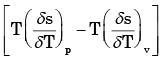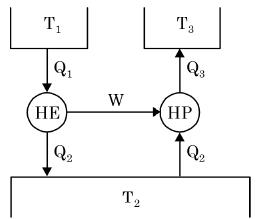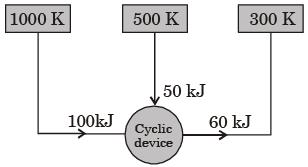Courses

# Past Year Questions: Second Law Of Thermodynamics, Carnot Cycle, And Entropy Notes | EduRev

## Mechanical Engineering : Past Year Questions: Second Law Of Thermodynamics, Carnot Cycle, And Entropy Notes | EduRev

The document Past Year Questions: Second Law Of Thermodynamics, Carnot Cycle, And Entropy Notes | EduRev is a part of the Mechanical Engineering Course Thermodynamics.
All you need of Mechanical Engineering at this link: Mechanical Engineering

Question 1:For an ideal gas with constant properties undergoing a quasi-static process, which one of the following represents the change of entropy (Δs) from state 1 to 2?

[2018, Set-2]

Question 2:Which one of the following pairs of equations describes an irreversible heat engine?

[2014, Set-3]

Question 3:An ideal gas of mass m and temperature T1 undergoes a reversible isothermal process from an initial pressure P1 to final pressure P2. The heat loss during the process is Q. The entropy change ΔS of the gas is



Question 4:One kilogram of water at room temperature is brought into contact with a high temperature thermal reservoir. The entropy change of the universe is



Question 5:If a closed system is undergoing an irreversible process, the entropy of the system



Question 6:A system undergoes a State change from 1 to 2. According to the second law of thermodynamics, for the process to be feasible, the entropy change, S2 – S1 of the system



Question 7:For an ideal gas the expressionis always equal to



Question 8:One kilomole of an ideal gas is throttled from an initial pressure of 0.5 MPa to 0.1 MPa. The initial temperature is 300 K. The entropy change of the universe is



Question 9:Which among the following relations is/are valid only for reversible process undergone by a pure substance?



Question 10:A Carnot cycle is having an efficiency of 0.75. If the temperature of the high temperature reservoir is 727°C. What is the temperature of low temperature reservoir?



Question 11:Consider a refrigerator and a heat pump working on the reversed Carnot cycle between the same temperature limits. Which of the following is correct?



Question 12:The figure shows a heat engine (HE) working between two reservoirs. The amount of heat (Q2) rejected by the heat engine is drawn by a heat pump (HP). The heat pump receives the entire work out put (W) of the heat engine. If temperatures, T1 > T3 > T2, then the relation between the efficiency (η) of the heat engine and the coefficient and the coefficient of performance (COP) of the heat pump is[2019, Set -2]

Question 13:A reversible heat engine receives 2 kJ of heat from a reservoir at 1000 K and a certain amount of heat from a reservoir at 800 K.It rejects 1 kJ of heat to a reservoir at 400 K.
The net work output (in kJ) of the cycle is

[2014, Set-1]

Question 14:Consider the following two processes;
I. A heat source at 1200 K loses 2500 kJ of heat to a sink at 800 K
II. A heat source at 800 K loses 2000 kJ of heat to a sink at 500 K
Which of the following statements is true?



Question 15:An irreversible heat engine extracts heat from a high temperature source at a rate of 100 kW and rejects heat to a sink at a rate of 50 kW.The entire work output of the heat engine is used to drive a reversible heat pump operating between a set of independent isothermal heat reservoirs at 17°C and 75°C. The rate (in kW) at which the heat pump delivers heat to its high temperature sink is



Question 16:A cyclic device operates between three thermal reservoirs, as shown in the figure. Heat is transferred to/from the cycle device. It is assumed that heat transfer between each thermal reservoir and the cyclic device takes place across negligible temperature difference.
Interactions between the cyclic device and the respective thermal reservoirs that are shown in the figure are all in the form of heat transfer.The cyclic device can be



Question 17:A heat transformer is device that transfers a part of the heat, supplied to it at an intermediate temperature, to a high temperature reservoir while rejecting the remaining part to a low temperature heat sink.In such a heat transformer, 100 kJ of heat is supplied at 350 K. The maximum amount of heat in kJ that can be transferred to 400 K, when the rest is rejected to a heat sink at 300 K is



Question 18:A solar collector receiving solar radiation at the rate of 0.6 kW/m2 transforms it to the internal energy of a fluid at an overall efficiency of 50%. The fluid heated to 350 K is used to run a heat engine which rejects heat at 313 K.If the heat engine is to deliver 2.5 kW power, the minimum area of the solar collector required would be



Question 19:A cycle heat engine does 50 kJ of work per cycle. If the efficiency of the heat engine is 75%. The heat rejected per cycle is



Question 20:For two cycles coupled in series, the topping cycle has an efficiency of 30% and the bottoming cycle has an efficiency of 20%. The overall combined cycle efficiency is



Question 21:A solar energy based heat engine which receives 80 kJ of heat at 100°C and rejects 70 kJ of heat to the ambient at 30°C is to be designed. The thermal efficiency of the heat engine is



Question 22:When a system executes an irreversible cycle



Question 23:A reversible heat transfer demands:



Question 24:A condenser of a refrigeration system rejects heat at a rate of 120 kW, while is compressor consumes a power of 30 kW. The coefficient of performance of the system would be



Offer running on EduRev: Apply code STAYHOME200 to get INR 200 off on our premium plan EduRev Infinity!

## Thermodynamics

56 videos|92 docs|33 tests

,

,

,

,

,

,

,

,

,

,

,

,

,

,

,

,

,

,

,

,

,

,

,

,

,

,

,

;